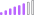cancel
Showing results for
Did you mean:

Fabric is Generally Available. Browse Fabric Presentations. Work towards your Fabric certification with the Cloud Skills Challenge.Post Patron

## 12week rolling average

Hi Experts

need to calculate the 12 week rolling average see sample data - PS i have all the necessary columns in my DimDate Table, i want to plot the 12 week rolling average in a graph

sample Data1 ACCEPTED SOLUTIONSolution Sage
_12wk Average =
VAR Week = CALCULATE(DISTINCTCOUNT('Date'[Week]),FILTER(
ALL('Date'),
(
(
'Date'[Week]
>= MAX ('Date'[Week] ) - 11
)
&& (
'Date'[Week]
<= MAX ( 'Date'[Week] ) - 0
)
)
))
VAR Total=CALCULATE(SUM('Table'[Sales]),
FILTER(
ALL('Date'),
(
(
'Date'[Week]
>= MAX ('Date'[Week] ) - 11
)
&& (
'Date'[Week]
<= MAX ( 'Date'[Week] ) - 0
)
)
))

RETURN
DIVIDE(Total,Week,12)Solution Sage
_12wk Average =
VAR Week = CALCULATE(DISTINCTCOUNT('Date'[Week]),FILTER(
ALL('Date'),
(
(
'Date'[Week]
>= MAX ('Date'[Week] ) - 11
)
&& (
'Date'[Week]
<= MAX ( 'Date'[Week] ) - 0
)
)
))
VAR Total=CALCULATE(SUM('Table'[Sales]),
FILTER(
ALL('Date'),
(
(
'Date'[Week]
>= MAX ('Date'[Week] ) - 11
)
&& (
'Date'[Week]
<= MAX ( 'Date'[Week] ) - 0
)
)
))

RETURN
DIVIDE(Total,Week,12)Announcements#### Power BI Monthly Update - November 2023

Check out the November 2023 Power BI update to learn about new features.#### The largest Power BI and Fabric virtual conference

130+ sessions, 130+ speakers, Product managers, MVPs, and experts. All about Power BI and Fabric. Attend online or watch the recordings.Top Solution Authors
Top Kudoed Authors
Users online (6,536)